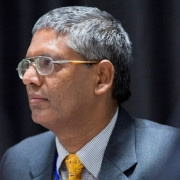Message from HODWelcome to the Central Department of Mathematics, Tribhuvan University! Mathematics is very interesting, important and forms the foundation of physical sciences and technology. Its importance is growing in various areas such as business, finance, management, engineering, ICT, biology, medicine, and many social sciences. The department was started in the same year as the establishment of Tribhuvan University in 1959 AD (2016 BS) and runs under the Institute of Science and Technology. It off...
Seminar/Workshop
Feb 27 2023
International Workshop in Computational Mathematics (IWCM-2023)
Conferences
Apr 11 2023
Third International Conference on Applications of Mathematics to Nonlinear Sciences (AMNS-2023)
Master's Level

Master’s Degree (MA/MSc) program, of 4 semesters duration, covers courses in pure and applied mathematics. The total credit requirement for the degree is 100 (25 per semester). The thesis writing of 5 credits for the fourth semester students is introduced as an optional subject. The focus is given to the depth rather than the breadth with the belief that anyone sufficiently versed in the rigorous techniques of mathematics can successfully move on to more applied and even to neighboring fields. For evaluation, in each semester, the department conducts internal assessments of 40 percent marks and Institute of Science and Technology conducts external examinations of 60 percent marks in each subject except for the thesis writing course. To pass an examination, a student must secure a minimum of 50 percent marks in each subject (separately for internal and external exams). The question papers, mainly, consist of subjective type covering conceptual understanding with examples, verifying statements and proving theorems parts. Currently, around 100 students (mostly from the colleges/campuses affiliated to Tribhuvan University) get admitted in Master’s program every year.

1. Math 551 Topology I
2. Math 552 Topology II
3. Math 553 Measure Theory and Integration I
4. Math 554 Functional Analysis I
5. Math 555 Complex Analysis and Applications I
6. Math 556 Differential Equations
7. Math 557 Theory of Functions I
8. Math 558 Theory of Functions II
9. Math 559 Differential Geometry
10. Math 560 Combinatorial Optimization
11. Math 561 Mathematical Programming
12. Math 562 Mathematical Analysis I
13. Math 563 Functions of Several Variables
14. Math 564 Elementary Harmonic Analysis
15. Math 565 Algebra I
16. Math 566 Algebra II
17. Math 567 Linear Algebra
18. Math 568 Linear Algebra and Its Applications
19. Math 569 Introduction to the Theory of Sets
20. Math 570 Theory of Numbers I
21. Math 571 Mechanics
22. Math 572 Functional Analysis II
23. Math 573 Harmonic Analysis
24. Math 574 Special Functions I
25. Math 575 Special Functions II
26. Math 576 Partial Differential Equations I
27. Math 577 Integral Transforms I
28. Math 578 Measure Theory and Integration II
29. Math 579 Scheduling Theory
30. Math 580 Integer Programming
31. Math 581 Network Optimization
32. Math 582 Graph Theory
33. Math 583 Numerical Analysis
34. Math 584 Numerical Optimization
35. Math 585 Dynamical Systems
36. Math 586 Dynamics of Viscous Fluids
37. Math 587 Partial Differential Equations II
38. Math 588 Introduction to C Programming
39. Math 589 Seminar
40. Math 590 Thesis
41. Math 591 Integral Transforms II
42. Math 592 Complex Analysis and Applications II
43. Math 593 Theory of Numbers II
44. Math 594 Scientific Computing
45. Math 595 Analysis of Finite Difference Scheme
46. Math 596 Introduction to Commutative Algebra
47. Math 597 Location Analysis
48. Math 598 Mathematical Model in Biology.
49. Math 599 Advanced Ordinary Differential Equations
Following files guide the thesis rules and thesis  writing. It is suggested to strictly follow them.

Master Thesis Rules(.pdf)
Master_Thesis_Template(.rar)

Master Thesis Application Form